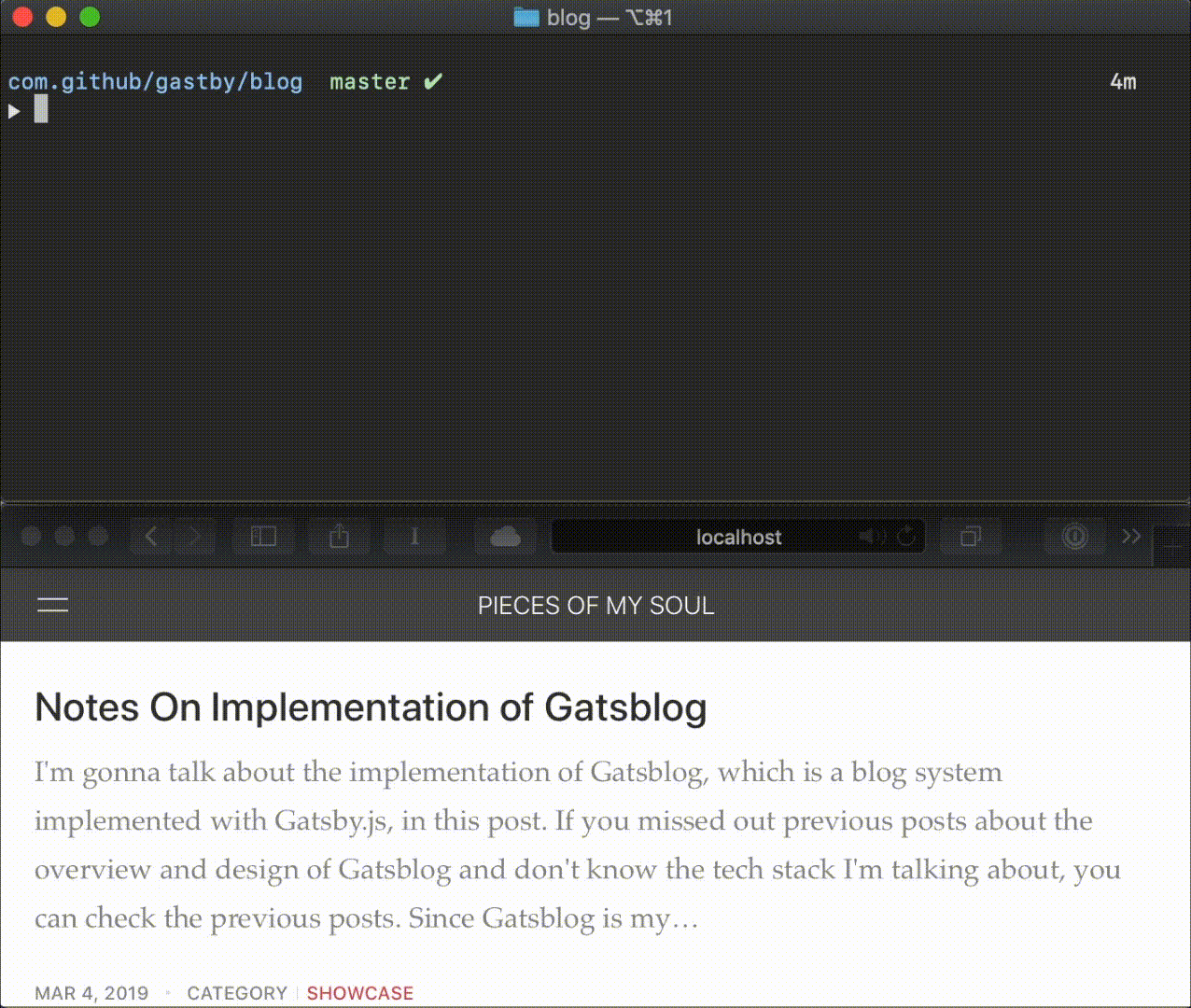# An Introduction to Gatsblog

## A Blog Built with Gatsby.js

As I mentioned in the Hello World post, there are no blog systems fulfill all my needs, time that I have such a deep touch with frontend tech stack.

Thus I got started to make my own one. And frankly, this is the first I just decided to write down the whole process, from design to implementation, to make a memento of my journey, which conducted by an iOS developer with industrial design background, into modern frontend tech stack.

## Pseudo-Live Editing

Any site built with Gatsby.js comes with a very naïve live editing feature. You can just launch Gatsby's develop mode server with gatsby develop -H 0.0.0.0 or npm run start and open a browser with address http://localhost:8000. Then you can watch your post changes each time you saved the edited file to the disk.## Sophisticated Grid System

I adopted a sophisticated grid system and tweaked the sizes of elements and the spaces between elements. The design is not very modern, but functional -- at least the content is clear and spaces between elements are not annoying.

## Responsive Image with Retina Display Support

Most of the figures on my posts are authored by myself. Thus it is very easy to output @2x and @3x version at the same time I'm outputting the original version. Thus Gatsby supports such a resolution density in file names. It recognizes image files with names like xxx.png, xxx@2x.png and xxx@3x.png as a series of image files whose resolution density is 1x, 2x and 3x. You only have to link your image with the image markup in Markdown grammar:

markdown![Image Alternative Text](xxx.png "Image Title")

Then Gatsblog handles responsiveness and Retina display support for you.

Since there are also images that we grabbed from web or taken by cameras asking users to offer versions for all the resolution densities is impossible. What happens to an image file without paired resolution density versions? Don't worry. If you just put an image file named after xxx.png in your Markdown document's folder, Gatsblog handles responsiveness support for you.

## KaTex

Because I'm a fan of math, Gatsby supports inline KaTex syntax like $$a^2 + b^2 = c^2$$ which rendered as ${a^2 + b^2 = c^2}$, and block KaTex syntax:

katex$$\frac{1}{\Bigl(\sqrt{\phi \sqrt{5}}-\phi\Bigr) e^{\frac25 \pi}} \equiv 1+\frac{e^{-2\pi}} {1+\frac{e^{-4\pi}} {1+\frac{e^{-6\pi}} {1+\frac{e^{-8\pi}} {1+\cdots} } } }$$
${\frac{1}{\Bigl(\sqrt{\phi \sqrt{5}}-\phi\Bigr) e^{\frac25 \pi}} \equiv 1+\frac{e^{-2\pi}} {1+\frac{e^{-4\pi}} {1+\frac{e^{-6\pi}} {1+\frac{e^{-8\pi}} {1+\cdots} } } }}$
katex$$\left( \sum_{k=1}^n a_k b_k \right)^2 \leq \left( \sum_{k=1}^n a_k^2 \right) \left( \sum_{k=1}^n b_k^2 \right)$$
${\left( \sum_{k=1}^n a_k b_k \right)^2 \leq \left( \sum_{k=1}^n a_k^2 \right) \left( \sum_{k=1}^n b_k^2 \right)}$
katex$$\int u \frac{dv}{dx}\,dx=uv-\int \frac{du}{dx}v\,dx$$
${\int u \frac{dv}{dx}\,dx=uv-\int \frac{du}{dx}v\,dx }$

## Enhanced with MDX

### React Live

class Counter extends React.Component {
constructor() {
super();
this.state = { count: 0 };
}

componentDidMount() {
this.interval = setInterval(() => {
this.setState(state => ({ count: state.count + 1 }));
}, 1000);
}

componentWillUnmount() {
clearInterval(this.interval);
}

render() {
return (
<center>
<h3>{this.state.count}</h3>
</center>
);
}
}

### Code Block with Path Label

By putting path=path_of_file after the language metadata in the beginning line of the fenced code block

markdownc path=src/main.c#include <stdio>int main(int argc, char[] * args) {    printf("Hello, world!\n");    return 0;}​​

you can get a code block likes

src/main.cc#include <stdio>int main(int argc, char[] * args) {    printf("Hello, world!\n");    return 0;}

## Statically Deployed

Empowered by Gatsby.js, Gatsblog can be statically deployed. You can deploy a site by several clicking on Netlify.

Freiheit ist Wille. Handeln ist Fähigkeit. Mut ist der Glaube, der mit der Zeit nicht nachlässt.
Built with Gatsby.js.